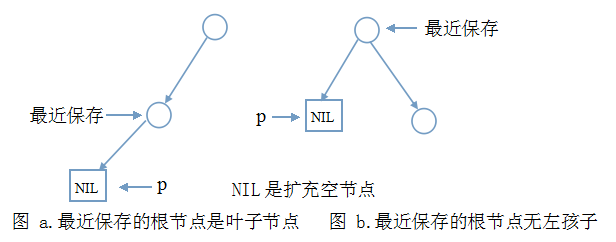# 二叉树前序、中序、后序遍历非递归写法的透彻解析

### 前言

//Binary Tree Node
typedef struct node
{
int data;
struct node* lchild;  //左孩子
struct node* rchild;  //右孩子
}BTNode;

### 中序遍历

#### 中序代码段(i)

BTNode* p = root;  //p指向树根
stack<BTNode*> s;  //STL中的栈
//一直遍历到左子树最下边，边遍历边保存根节点到栈中
while (p)
{
s.push(p);
p = p->lchild;
}1. 上图中只给出了必要的节点和边，其它的边和节点与讨论无关，不必画出。
2. 你可能认为图a中最近保存节点算不得是根节点。如果你看过树、二叉树基础，使用扩充二叉树的概念，就可以解释。总之，不用纠结这个没有意义问题。
3. 整个二叉树只有一个根节点的情况可以划到图a。

p = s.top();
s.pop();
cout << p->data;

1.图a中访问的是一个左孩子，按中序遍历顺序，接下来应访问它的根节点。也就是图a中的另一个节点，高兴的是它已被保存在栈中。我们只需这样的代码和上一步一样的代码：
p = s.top();
s.pop();
cout << p->data;

2.再看图b，由于没有左孩子，根节点就是中序序列中第一个，然后直接是进入右子树：p=p->rchild;在右子树中，又会新一轮的代码段(i)、代码段(ii)……直到栈空且p空。

p = s.top();
s.pop();
cout << p->data;
p = s.top();
s.pop();
cout << p->data;
p = p->rchild;

p = s.top();
s.pop();
cout << p->data;
p = p->rchild;

#### 中序代码段(ii)

p = s.top();
s.pop();
cout << p->data;
p = p->rchild;


BTNode* p = root;
stack<BTNode*> s;
while (!s.empty() || p)
{
//代码段(i)一直遍历到左子树最下边，边遍历边保存根节点到栈中
while (p)
{
s.push(p);
p = p->lchild;
}
//代码段(ii)当p为空时，说明已经到达左子树最下边，这时需要出栈了
if (!s.empty())
{
p = s.top();
s.pop();
cout << setw(4) << p->data;
//进入右子树，开始新的一轮左子树遍历(这是递归的自我实现)
p = p->rchild;
}
}

#### 中序遍历代码一

//中序遍历
void InOrderWithoutRecursion1(BTNode* root)
{
//空树
if (root == NULL)
return;
//树非空
BTNode* p = root;
stack<BTNode*> s;
while (!s.empty() || p)
{
//一直遍历到左子树最下边，边遍历边保存根节点到栈中
while (p)
{
s.push(p);
p = p->lchild;
}
//当p为空时，说明已经到达左子树最下边，这时需要出栈了
if (!s.empty())
{
p = s.top();
s.pop();
cout << setw(4) << p->data;
//进入右子树，开始新的一轮左子树遍历(这是递归的自我实现)
p = p->rchild;
}
}
}

#### 中序遍历代码二

//中序遍历
void InOrderWithoutRecursion2(BTNode* root)
{
//空树
if (root == NULL)
return;
//树非空
BTNode* p = root;
stack<BTNode*> s;
while (!s.empty() || p)
{
if (p)
{
s.push(p);
p = p->lchild;
}
else
{
p = s.top();
s.pop();
cout << setw(4) << p->data;
p = p->rchild;
}
}
}

### 前序遍历

#### 前序代码段(i)

//边遍历边打印，并存入栈中，以后需要借助这些根节点(不要怀疑这种说法哦)进入右子树
while (p)
{
cout << setw(4) << p->data;
s.push(p);
p = p->lchild;
}

#### 前序代码段(ii)

//当p为空时，说明根和左子树都遍历完了，该进入右子树了
if (!s.empty())
{
p = s.top();
s.pop();
p = p->rchild;
}

#### 前序遍历代码一

void PreOrderWithoutRecursion1(BTNode* root)
{
if (root == NULL)
return;
BTNode* p = root;
stack<BTNode*> s;
while (!s.empty() || p)
{
//边遍历边打印，并存入栈中，以后需要借助这些根节点(不要怀疑这种说法哦)进入右子树
while (p)
{
cout << setw(4) << p->data;
s.push(p);
p = p->lchild;
}
//当p为空时，说明根和左子树都遍历完了，该进入右子树了
if (!s.empty())
{
p = s.top();
s.pop();
p = p->rchild;
}
}
cout << endl;
}

#### 前序遍历代码二

//前序遍历
void PreOrderWithoutRecursion2(BTNode* root)
{
if (root == NULL)
return;
BTNode* p = root;
stack<BTNode*> s;
while (!s.empty() || p)
{
if (p)
{
cout << setw(4) << p->data;
s.push(p);
p = p->lchild;
}
else
{
p = s.top();
s.pop();
p = p->rchild;
}
}
cout << endl;
}

#### 前序遍历代码三

void PreOrderWithoutRecursion3(BTNode* root)
{
if (root == NULL)
return;
stack<BTNode*> s;
BTNode* p = root;
s.push(root);
while (!s.empty())  //循环结束条件与前两种不一样
{
//这句表明p在循环中总是非空的
cout << setw(4) << p->data;
/*
栈的特点：先进后出
先被访问的根节点的右子树后被访问
*/
if (p->rchild)
s.push(p->rchild);
if (p->lchild)
p = p->lchild;
else
{//左子树访问完了，访问右子树
p = s.top();
s.pop();
}
}
cout << endl;
}

### 后序遍历

#### 后序遍历代码一

//后序遍历
void PostOrderWithoutRecursion(BTNode* root)
{
if (root == NULL)
return;
stack<BTNode*> s;
//pCur:当前访问节点，pLastVisit:上次访问节点
BTNode* pCur, *pLastVisit;
//pCur = root;
pCur = root;
pLastVisit = NULL;
//先把pCur移动到左子树最下边
while (pCur)
{
s.push(pCur);
pCur = pCur->lchild;
}
while (!s.empty())
{
//走到这里，pCur都是空，并已经遍历到左子树底端(看成扩充二叉树，则空，亦是某棵树的左孩子)
pCur = s.top();
s.pop();
//一个根节点被访问的前提是：无右子树或右子树已被访问过
if (pCur->rchild == NULL || pCur->rchild == pLastVisit)
{
cout << setw(4) << pCur->data;
//修改最近被访问的节点
pLastVisit = pCur;
}
/*这里的else语句可换成带条件的else if:
else if (pCur->lchild == pLastVisit)//若左子树刚被访问过，则需先进入右子树(根节点需再次入栈)
因为：上面的条件没通过就一定是下面的条件满足。仔细想想！
*/
else
{
//根节点再次入栈
s.push(pCur);
//进入右子树，且可肯定右子树一定不为空
pCur = pCur->rchild;
while (pCur)
{
s.push(pCur);
pCur = pCur->lchild;
}
}
}
cout << endl;
}

#### 后序遍历代码二

//定义枚举类型：Tag
enum Tag{left,right};
//自定义新的类型，把二叉树节点和标记封装在一起
typedef struct
{
BTNode* node;
Tag tag;
}TagNode;
//后序遍历
void PostOrderWithoutRecursion2(BTNode* root)
{
if (root == NULL)
return;
stack<TagNode> s;
TagNode tagnode;
BTNode* p = root;
while (!s.empty() || p)
{
while (p)
{
tagnode.node = p;
//该节点的左子树被访问过
tagnode.tag = Tag::left;
s.push(tagnode);
p = p->lchild;
}
tagnode = s.top();
s.pop();
//左子树被访问过，则还需进入右子树
if (tagnode.tag == Tag::left)
{
//置换标记
tagnode.tag = Tag::right;
//再次入栈
s.push(tagnode);
p = tagnode.node;
//进入右子树
p = p->rchild;
}
else//右子树已被访问过，则可访问当前节点
{
cout << setw(4) << (tagnode.node)->data;
//置空，再次出栈(这一步是理解的难点)
p = NULL;
}
}
cout << endl;
}<span style="font-family: 'Courier New'; ">  </span>

### 总结

1. 所有的节点都可看做是父节点(叶子节点可看做是两个孩子为空的父节点)。
2. 把同一算法的代码对比着看。在差异中往往可看到算法的本质。
3. 根据自己的理解，尝试修改代码。写出自己理解下的代码。写成了，那就是真的掌握了。

09-143万+10-212万+
05-2312万+
06-0821万+
07-097256
03-112万+
01-162万+
05-15349
06-032万+
06-173307
05-0739万+
10-255万+
05-216万+
10-163万+
10-244万+
04-288万+
12-3078
09-22997
04-1092